Refer to our Texas Go Math Grade 7 Answer Key Pdf to score good marks in the exams. Test yourself by practicing the problems from Texas Go Math Grade 7 Lesson 13.3 Answer Key Making Purchasing Decisions.

Example 1.
Silvie went to a local warehouse store to buy her family’s favorite sports drink. Which of the two options described below is the better buy?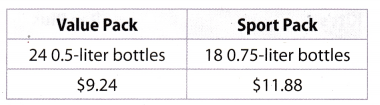Step 1
Find the total amount of the sports drink in each purchase.
Value Pack: 24(0.5) = 12 liters
Sport pack: 18(0.75) = 13.5 liters
Step 2
Find each unit price.The Value Pack is a better buy, because $0.77 <$0.88.

Question 1.
Financial Literacy Manny must choose between a pack of four 6.5-ounce containers of yogurt for $3.77 or three 8-ounce containers at$1.29 each. Which ¡s the better buy? Why?
Find the total. amount of the yogurt in each purchase.
A pack of 46.5 – ounce containers
4 × 6.5 = 26 – ounces
A pack of 38- ounce containers
3 × 8 = 24 – ounces

Now, find each unit price.
A pack of 46.5-ounce containers
$$\frac{\ 3.77}{26-\text { ounces }}$$ = $0.145 per ounce A pack of 38-ounce containers Each 8—ounce container costs$1.29. hence the price of 3 containers is
3 × $1.29 =$3.87.
$$\frac{\ 3.87}{24-\text { ounces }}$$ = $0.16 per ounce Better buy is a pack of 4 6.5— ounce containers, because$0.145 < $0.16 Example 2 Nathan plans on hiking this summer and wants to buy a solar charger for his cell phone and notebook computer. He finds the charger that he wants at two stores. Which store has the lower price for the charger?Answer: Step 1 Find the discount price at each store. Kitt’s discount price = regular price – discount =$89.99 – 0.3($89.99) =$89.99 – $26,997 =$62,993
To the nearest cent, the discount price at Kitti’s is $62.99 Rec Plus discount price = regular price – discount =$79.99 – $15.00 =$64.99
The discount price at Rec Plus is $64.99. Step 2 Compare the discounted prices. Kitt’s offers the lower price, because$62.99 < $64.99. Reflect Question 2. Critical Thinking Why might Nathan choose the higher priced item? Answer: Nathan would choose the charger in Kitt’s even if it has a higher regular price. This is because the discount in Kitt’s is higher compared to the Rec Plus. Once the regular price for the charger in Kitt’s got discounted, the price is cheaper compared to the discounted price in Rec Plus. The discount is higher for the higher-priced item which made it cheaper after the discount Your Turn Question 3. Nathan finds a backpack at Kitt’s that is regularly$84.99, but is on sale for $$\frac{1}{3}$$ off. The same backpack is regularly $72.99 at Rec Plus but is on sale for 25% off. Which is a better buy? Explain. Answer: Find the discount price at each store. Kitts Discount price = Regular price – Discount To find the Discount, multiply the Regular price by the percent of discount.$84.99 × $$\frac{1}{3}$$ = $28.33 Discouut price =$84.99 – $28.33 Discount price =$56.66
The Discount price at Kitt’s is $56.66. Rec Plus Discount price = Regular price – Discount To find the Discount multiply the Regular price by the percent of discount. Write percent as decimal, ie 25% = 0.25.$72.99 × 0.25 = $18.25 Discount price =$72.99 – $18.25 Discount price =$54.74
Tue Discount price at Rec Plus is $54.74. Rec Plus offers the lower price, because$54.74 <$56.66. Example 3. Cedelia can get the same running Go shoes at two different stores. She wants to get two pairs of the same style. Which is the better buy?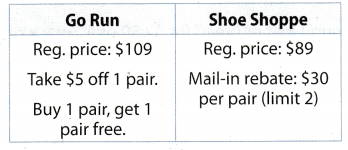Go Run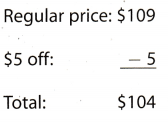She pays$104 for 2 pairs.
Shoe ShoppeShe pays 2 × $59 =$118 for 2 pairs
Go Run has the better buy because $104 <$118.

Reflect

Question 4.
What If? Suppose the total price at Shoe Shoppe had been $103? What are some conditions of a rebate that would make it a better decision to buy the shoes at Go Run? Answer: First condition If the first pair has rebate at least$7 and you get second pair free.
Proof:
Regular price is $109, rebate is at least$7, hence the total price at Go Run is $109 —$7 = $102.$102 < $103 Hence, Go Run lias the better buy than Shoe Shoppe. Second condition If both pairs have a discount of 53%. Proof: The regular price is$109.
Discount price = Regular price – Discount
To find the Discount multiply the Regular price by the percent of discount.
Write percent as decimal, ie 53% = 0.53.
$109 × 0.53 =$57.77

Discount price = $109 –$57.77
Discount price = $51.23 The Discount price of one pair is$51.23, and the discount price of 2 pairs is
$51.23 × 2 =$102.16
$102.46 <$103
Hence, Go Run has the better buy than Shoe Shoppe.

First condition: If the first pair has rebate at least $7 and you get second pair free. Second condition: If both pairs have a discount of 53%. Your Turn Question 5. Greta wants to buy 3 jars of honey. Food Mart sells it for$8.99 with a “Buy 2, get 1 free” offer. Leona’s sells it for $8.49 with a coupon for 50% off with a limit of 2 coupons. Which store has the better deal? Answer: Food Mart Greta wants to buy 3 jars of honey, and Food Mart lias an offer if you buy 2 you get 1 free. Hence, Greta will buy 2 jars of honey and get one free, and that would be 3 jars of honey she needs. One jar costs$8.99. so
Price of 2 jars = 2 × $8.99 =$17.98
Greta would pay $17.98 for 3 jars of honey at Food Mart. Leona‘s At Leon’s she can buy 2 jars with a coupon for 50% off, because there is a limit of 2 coupons. Hence, the third jar of honey she has to buy by the full price. One jar of honey costs$8.19.
Let’s find the Discount price with a coupon.
Discount price = Regular price – Discount
To find the Discount multiply the Regular price by the percent of discount.
Write percent as decimal, ie 50% = 0.5.
$8.19 × 0.5 =$4.215
Discount price = $8.49 –$4.245
Discount price = $4.245 The Discount price of one jar of honey is$4.245.
She would buy 2 jars of honey by the discount price and the third one by the full price.
Hence
Price of 3 jars = 2 × Discount price + Full price
Price of 3 jars = 2 × $4.245 +$8.49
Price of 3 jars = $16.98 Greta would pay$16.98 for 3 jars of honey at Leona’s.
Hence, Leon’s has the better buy, because $16.98 <$17.98.

Question 1.
The Corner Store sells 4 bottles of energy drink for $1 1.56. Bev’s sells 6 bottles for$17.94. Which is the better buy? (Example 1)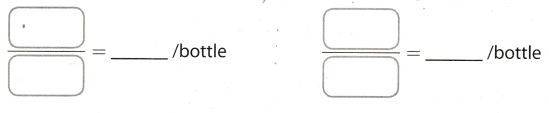Find the price of one energy drink in each purchase.
The Corner Store
4 bottles of energy drink cost $11.56. Find the price of one bottle of energy drink. Price of one bottle = $$\frac{\ 11.56}{4}$$ =$2.89/bottle
The price of one bottle at Coriier Store is $2.89 per bottle. Bev’s 6 bottles of energy drink cost$17.94.
Find the price of one bottle of energy drink.
Price of 0ne bottle = $$\frac{\ 17.94}{6}$$ = $2.99/bottle The price of one bottle at Bev’s is$2.99 per bottle.
The Corner Store lias the better buy, because $2.89 <$2.99.

The Corner Store has the better buy, because $2.89 <$2.99.

Question 2.
You can buy a 2-pound loaf of bread for $2.50 or a 2-pack of 1$$\frac{1}{2}$$-pound loaves for$3.30. Which is the better buy?(Example 1)
Find the price of 0ne pound loaf of bread in each purchase.
First purchase
2-pound loaf of bread for 82.50
Find the price of one pound loaf of bread.
Price of one pound = $$\frac{\ 2.50}{2}$$ = $1.25 The price of one pound of loaf of bread is$1.25.
Second purchase
2- pack of 1$$\frac{1}{2}$$– pound loaf of bread for $3.30 In this purchase we have 2-pack of 4$$\frac{1}{2}$$ = $$\frac{3}{2}$$ = 1.5- pound loaves. Hence, the whole purchase will be 2 × 1.5 =3. So, 3- pound loaf of bread costs$3.30.
Find the price of one pound loaf of bread.
Price of one pound = $$\frac{\ 3.30}{3}$$ = $1.1 The price of one pound of loaf of bread is$1.1.
Second puchase is the better, because $1.1 <$1.25.

Question 3.
Marta can buy a 6-pack of 15-ounce cans of broth for $11.29 or three 32-ounce containers for$10.97. Which is the better buy? (Example 1)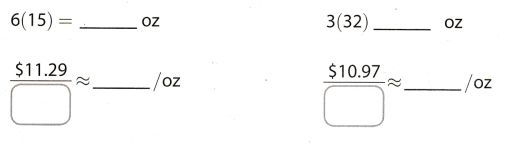First purchase
A 6- pack of 15- ounce cans of broth
6(15) = 90 oz
Now, find unite price
$$\frac{\ 11.29}{90-\mathrm{oz}}$$ = $0.125/oz Second purchase A 3-32- contains 3(32) = 96 oz Now, find unite price $$\frac{\ 10.97}{96-\text { ounces }}$$ =$0.11/oz
Second purchase is the better, because $0.11 <$0.125.

Question 4.
Zena can get an item online for $19 that weighs 8 pounds. Shipping and handling costs$0.49 per pound. She can also buy the same item locally for $26.95, and she has a$5 off coupon. Which is the better buy? (Example 2)
The item has 8 pounds and shipping and handling per pound is $0.49. Shipping and handling for item is$0.49 × 8 = $3.92 To find the whole price of item sum the online price and the cost of shipping and handling.$19 + $3.92 =$22.92
Online purchase costs $22.92. Locally purchase$5 off coupon is a Discount.
Find the Discount price.
Discount price = Regular price — Discount
Discount price = $26.95 —$5
Discount price = $21.95 The Discount price for locally purchase is$21.95.
The locally purchase is better, because $21.95 <$22.92.

Question 5.
A take-and-bake pizza chain has several coupons, which cannot be used together. J.D. is buying a pizza that costs $17.90. Should he use a$5 off coupon or a 35% off coupon? (Example 2)
$5 off coupon$5 off coupon is a Discount.
Find the Discount price.
Discount price = Regular price – Discount
Discount price = $17.90 –$5
Discount price = $12.90 The Discount price of a pizza with a$5 off coupon is $12.90. 35% off coupon Discount price = Regular price – Discount To find the Discount multiply the Regular price by the percent of discount. Write percent as decimal, ie 35% = 0.35.$17.90 × 0.35 = $6.26 Discount price =$17.90 – $6.26 Discount price =$11.64
The Discount price of a pizza with a 35% coupon off is $11.64. J.D. should use a 35% off coupon, because$11.61 <$12.90. Question 6. The King family buys an HDTV selling for$825 at PanView with a 20% off coupon and a rebate of $125. They later see the TV on sale at Rey’s TVs for$555. Did they get the better deal? (Example 3)
Determine the cost of the HDTV the King fami[y purchased at PanView. Show the 20% off coupon as decimaL
825 × 0.20 = 165 Determine the discount for the HDTV
825 – 165 = 660 Subtract the discount from the original price
660 – 125 = 535 Subtract the rebate from the discounted price
The King family get the better deal. purchasing the HDTV at PanView instead at Rey’s TVs
Yes

Question 7.
Credit card A has an annual fee of $50 but gives you a rebate at the end of the year of 2% of your total purchases. Credit card B has no annual fee or rebate. If you spend about$150 a month, which card is better for you? (Example 3)
Credit card A
If you spend $150 a month, it means that you spend 12 ×$150 = $1,800 a year. Credit card A has an annual fee of$50, hence, we have to add fee to the amount you spend a year.
$1,800 +$50 = $1,850 At the end of the year you get a rebate of 2% of your total purchase, hence we have to subtract 2% from the whole amount$1,850.
Write percent as decimal, ie 2% = 0.02.
Find 2% of $1,850.$1,850 × 0.02 = $37 Now subtract.$1,850 – $37 =$1,813
If you use Credit card A, you will spend $1,813 a year. Credit card B Credit card B has no annual or rebate, hence, yearly you will spend 12 ×$150 = $1,800 If you use Credit card B, you will spend$1,800 a year.

Hence, the Credit card B is better, because $1,800 <$1,813.

Essential Question Check-In

Question 8.
Is the lower unit price always the best buy?
No, it is not always.
Sometimes there is an offer like, “Buy 2, get 1 free” of items with higher unit price.
Also, there is some discount on quantity on items with higher unit price, which could be better buy than buy of unit with lower unit price without discount.
The quality of the product when you buy it, regardless of the price, should not be neglected.

Question 9.
Store 1 has a price of $99 on an MP3 player. Store 2 is offering the same MP3 player at a sale price of 25% off their regular price of$125. Jerusha wants to buy an MP3 player. From which store should she buy it? Explain.
Store 2
Discount price = Regular price – Discount
To find the Discount multiply the Regular price by the percent of discount.
Write percent as decimal, ie 25% = 0.25.
Discount = $125 × 0.25 =$31.25
Discount price $125 –$31.25
Discount price = $93.75 The Discount price of an MP3 player in Store 2 is$93.75.

The price of an MP3 player in Store 1 is $99. He should buy an MP3 player in Store 2, because$93.75 < $99. Question 10. Multistep Randy wants trail mix for his hiking trip. The ready-made trail mix costs$8.95 for 1.5 pounds. The costs of the bulk ingredients to make one pound of trail mix are as follows: salted peanuts—$1.25, raisins—$1.70, sunflower seeds—$0.50, cashews—$0.87, and almonds—$0.65. If Randy plans to take 6 pounds of trail mix, which option is cheaper? How much does he save by choosing the cheaper option? Answer: The ready-made trail mix A pack of 1.5 pounds costs$8.95. and Randy wants to buy 6 pounds of it.
Hence, he needs to buy 1 packs of 1.5 pounds of trail mix.
4 × $8.95 =$35.8
6 pounds of ready-made trail mix will cost $35.8. Self—made trail mix To find the cost of one pound of trail mix. prices of all ingredients should be summed up. One pound of self-made trail mix =$1.25 + $1.70 +$0.50 + $0.87 +$0.65 = $4.97 To find the cost of 6 pounds of self-made trail mix multiply by 6 the cost of one pound. 6 ×$4.97 = $29.82 The cost of 6 pounds of self-made trail mix will be$29.82.

The self-made trail mix is cheaper option than the ready—made trail mix, because $29.82 <$35.8.

Question 11.
Tyron and Penelope both bought 4-wheelers. Tyron paid $199 upfront, and will pay the remainder in 12 payments of$50 each. Penelope paid nothing up front, and will make 18 payments of $49. Who got the better buy? Explain. Answer: Tyron To find how much Tyron paid 4—wheeler, sum the amount he paid up front and the total remainder. First, find the total remainder. To find the total remainder multiply the number of payments by the amount of each payment. The total remainder = 12 ×$50 = $600 Now, find the total cost. The total cost of 4—wheeler =$199 + $600 =$799
Tyron paid $799 for 4—wheeler. PeneloPe Unlike Tyron Penelope will pay the price in 18 payments of$49.
To find the total cost multiply the number of payments by the amount of each palyment.
The total cost of 4—wheeler = 18 × $19 =$882
Penelope paid $882 for 4—wheeler. Tyron lias the better buy that Penelope, because$799 < $882. Question 12. Financial Literacy T’Shonda is buying a laptop computer. She will pay 8% sales tax on the price before ány rebates. Where will she get a better buy? Explain.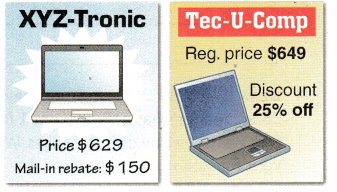Answer: XYZ-Tronic First, find the sales tax on the price(Subtotal). Write the tax rate as decimal, ie 8% = 0.08 Tax = Subtotal × Tax rate Tax =$629 × 0.08 = $50.32 Now, add the subtotal and the tax to find the total price. Total price =$629 + $50.32 =$679.32
The Total price before rebate is $679.32 To find the price after rebate, subtract mail—in—rebate from the Total price. The price after rebate =$679.32 – $150 =$529.32
In XYZ-Tronic she would pay $529.32 for a laptop. Tec U—Comp First, find the sales tax on the price(Subtotal). Write the tax rate as decimal. ie 8% = 0.08 Tax = Subtotal × Tax rate Tax =$649 × 0.08 = $51.92 Now, add the subtotal and the tax to find the total price. Total price =$649 + $51.92 =$700.92
The total price before rebate is $700.92 To find the price after rebate(The Discount price), subtract the Discount of 25% from the Total price(Regular price). Discount price = Regular price — Discount To find the Discount multiply the Regular price by the percent of discount. Write percent as decimal, ie 25% = 0.25. Discount =$700.92 × 0.25 = $175.23 Discount price =$700.92 – $175.23 Discount price =$525.69
The Discount price of a laptop is $525.69. T’Shonda will get the better buy in Tec—U-Comp, because$525.69 < $529.32. Question 13. Critical Thinking What single discount is equal to a discount of $$\frac{1}{4}$$ off followed by an additional 10% off the sale price? Answer: We want to know what single discount is equal to these discounts together. First, there is a discount of 25%. Write a fraction as decimal, ie $$\frac{1}{4}$$ = 0.25 Now, write the decimal as percent, ie 0.25 = 25% Hence, when some item has discount of $$\frac{1}{4}$$, it means that item has discount of 25%. After the discount of 25%, the total cost of that item is actually 75% of the regular price(the regular price represents 100% of the item) of that item, because 100% – 25% = 75%. After the discount of 25%, there is an additional. discount of 10%. An additional discount of 10% we can not compute on 100% of item price, because now the actual cost of the item is 75% of the first regular price(because first we had a discount of 25%). So, now we have to find 10% of 75% and subtract from it Write percent as decimal, ie 10% = 0.1 To find 10% of 75%, multiply 75% by the percent written in decimal. 75% × 0.1 = 7.5% Now, subtract. 75% – 7.5% = 67.5% Finally, the total cost of the item with first discount of 25% and then an additional 10% is 67.5% of the reguLar price of that item. As the cost of an item is now 67.5%, this means that these two discounts together represent a 32.5% discount, because 100% – 67.5% = 32.5%. Hence, the single discount that is equal to a discount of $$\frac{1}{4}$$ off followed by an additional 10% off is 32.5% off. The single discount that is equal to a discount of $$\frac{1}{4}$$ off followed by an additional 10% off is 32.5% off. Question 14. Make a Conjecture John is comparing the prices of two bags of the same cereal. He notices that the larger bag holds 10 more ounces and costs$1.50 more. How can he use the unit price of the smaller bag to decide the better buy? Explain.
John can use the unit price of the smaller bag by multiplying it by 10 since the larger bag contains 10 more ounces. If the result of this is more than $1.50, then it is better to buy the larger bag instead of the smaller bag. But if the result is less than$1.50, it is still better to buy the smaller bag.

Multiply the unit price of the smaller bag by 10.

Texas Go Math Grade 7 Lesson 13.3 H.O.T. Focus on Higher Order Thinking Answer Key

Question 15.
Draw Conclusions Mr. Jaros has the following options for buying a digital video recorder and access to the recording service for 2 years.
DiV: $49.99 digital video recorder, 2-year subscription at$16.98 a month
TVU: Free digital video recorder, 2-year commitment at $19.95 a month a. Which is the better deal? Explain. Answer: DiV To find the total cost. of a digital video recorder and access to the recording service for 2 years we have to sum the cost of digital video recorder and the total cost of 2—year subscription. To find the total cost of subscription multiply the number of months by monthly fee for subscription. One year have 12 months, and 2 years have 2 × 12 = 24 months. The total cost of subscription = 24 ×$16.98 = $407.52 Now find the total cost of a digital video recorder and access to the recording service for 2 years.$49.99 + $407.52 =$457.51
The offer at DiV would cost $457.51. TVU At TVU Mr.Jaros can get free digital video recorder and only would pay 2- year commitment at$19.93.
So, the total cost of a digital video recorder and access to the recording service for 2 years is actually the cost of total 2—years commitment.
To find the total cost of commitment multiply the number of months by monthly fee.
One year has 12 months, and 2 years have 2 × 12 = 24 months.
The total cost of commitment = 24 × $19.95 =$478.8
The offer at TVU would cost $478.8. The better deal is at DiV, because$457.51 < $478.8. b. What If? Suppose each offer were for 1 year. Would that change your answer? Explain. Answer: Div To find the total cost of a digital video recorder and access to the recording service for 1 years we have to sum the cost of digital video recorder and the total cost of 1-year subscription. To find the total cost of subscription multiply the number of months by monthly fee for subscription. One year has 12 months. The total cost of subscription = 12 ×$16.98 = $203.76 Now find the total cost of a digital video recorder and access to the recording service for 1 years.$49.99 + $203.76 =$253.75
The offer at DiV would cost $253.75. TVU At TVU Mr.Jaros can get free digital video recorder and only would pay 1-year commitment at$19.95.
So, the total cost of a digital video recorder and access to the recording service for 1 years is actually the cost of total 1-years commitment,
To find the total cost of commitment multiply the number of months by monthly fee.
One year has 12 months.
The total cost of commitment = 12 × $19.95 =$239.4
The offer at TVU would cost $239.4. If the offer were for 1 year, the better deal would be at TVU, because$239.4 < $253.75. c. Make a Conjecture Find the difference in monthly rates. Divide the cost of the DiV digital video recorder by the difference and round up to the nearest whole number. What does this quotient represent? Answer: The difference in monthly rates =$19.95 = $16.98 =$2.97
Now, divide the cost of the DiV digital video recorder by the difference we calculated.
$$\frac{49.99}{2.97}$$ = 16.83 ≈ 17
This quotient respresent the number of months.
If the offer were for this number of months, both offers would cost almost the same(because we round up the
quotient to the nearest whole number).
Proof:
DiV
The total cost of subscription = 17 × $16.98 =$288.66
To find the total cost of a digital video recorder and access to the recording service for 17 months we have to sum
the cost of digital video recorder and the total cost of 17 months subscription.
$49.99 +$288.66 = $338.65 TVU To find the total cost of commitment multiply the number of months by monthly fee. The total cost of commitment = 17 ×$19.95 = $339.15$338.65 ≈ $339.15 This quotient respresent the number of months. If the offer were for this number of months. Both offers would cost almost the same. Question 16. Analyze Relationships Sandy wants to buy 5 pounds of apples. She has a coupon for$1.00 off for every 3 pounds of apples. She gets to the store and discovers that apples are on sale for $0.75 a pound. Is it cheaper for her to buy 5 pounds or 6 pounds? Explain. Answer: If Sandy buys 5 pounds of apples. she can use only one coupon for$1 off, because the coupon is valid for every 3 pounds.
The cost of 011e pound of apples is $0.75. Hence, 5 pounds of apples. without coupon, will cost 5 ×$0.75 = $3.75 Now, to find the cost of 5 pounds of apples with a coupon. subtract$1 from $3.75.$3.75 – $1 =$2.75
Hence, 5 pounds of apples will cost $2.75 If Sandy buys 6 pounds of apples. she can use only two coupon for$1 off, because the coupon is valid for every 3 pounds.
The cost of 0ne pound of apples is $0.75. Hence, 6 pounds of apples, without coupon, will cost 6 ×$0.75 = $4.5 Now, to find the cost of 6 pounds of apples with two coupon, subtract$2(because she can use 2 coupons) from $4.5.$4.5 – $2 =$2.5
Hence, 6 pounds of apples will cost $2.5 It is cheaper for Sandy to buy 6 pounds of apples. because$2.5 <$2.75. Question 17. Draw Conclusions Two stores have a sale on T-shirts originally priced at$8.50. Yeager’s has a “Buy 2, get 1 free” sale. Gample’s has a 30% off sale. Dirk wants to buy only 2 T-shirts. Which is the better buy for him? Explain your reasoning.
Yeager ‘s
Yeager’s offer is “3 T-shirts for the price of 2”, ie “buy 2, get 1 free”.
Dirk wants to buy only 2 T-shirts, but buying 2 T-shirts will definitely get a third one.
Let ‘s see how much those 3 shirts would cost.
The price of 1 T-shirt is $8.50, so Dirk would pay for 2 T-shirts, and get a third one for free. The price of 3 T-shirts = 2 ×$8.50 = $17 3 T-shirts would cost$17, but we want to know what is the value of 2 T-shirts.
To find that, divide $17 by 3. The value of one T-shirt, = $$\frac{\ 17}{3}$$ =$5.67
Time value of 2 T-shirts = 2 × $5.67 =$11.34
The value of 2 T-shirts at Yeager’s is $11.34. Gample’s Gamples offer is a discount of 30% on each T—shirt. Let’s find how much one T-shirt would cost, ie the Discount price. Discount price = Regular price – Discount To find the Discount multiply the Regular price by the percent of discount. The regular price of 1 T-shirt is$8.50.
Write percent as dechnal, ie 30% = 0.30.
Discount = $8.50 × 0.30 =$2.55
[)iscount price = $8.50 –$2.55
Discount price = $5.95 The Discount price of one T-shirt is$5.95.
The Discount price(the value) of 2 T-shirts at Cample’s is 2 × $5.95 =$11.9.

Hence, the better buy for Dirk is at Yeager’s, because the value of 2 T-shirts is less than the value at Gample’s, ie $11.34 <$11.9.

The better buy for Dirk is at Yeager’s, because $11.34 <$11.9.

Question 18.
Represent Real-World Problems Suppose you are buying books from an online store, and the total price of your books is $39. Orders of$50 or more for eligible items qualify for free shipping. Would you buy more to qualify for free shipping? Or would you check out with only the books you have in your shopping cart? Explain your answer.
The first question that would come into your mind is, how much is the shipping fee for the books with a total price of $39. If the shipping fee is more than$11, it is better to buy another book which will make the total price at $50. But if the shipping fee is less than$11, lets say $5 and you really don’t have any book to purchase, then you could just pay the shipping fee and your total price will still be less than$50.